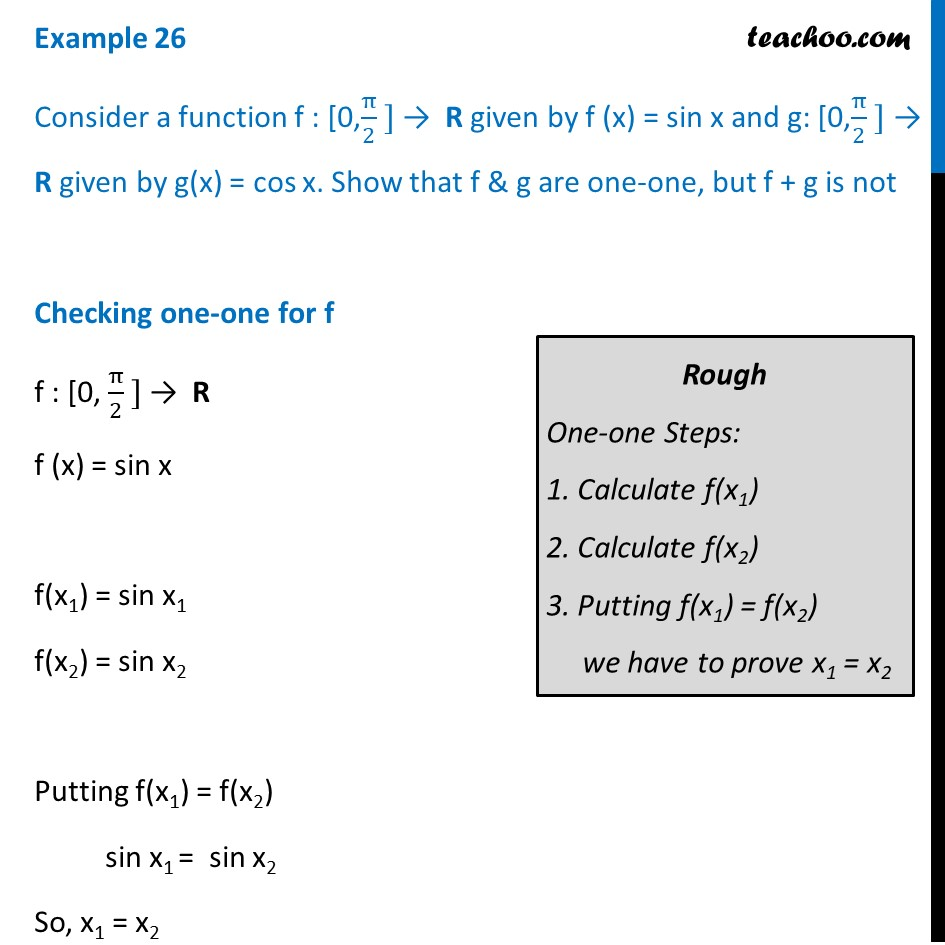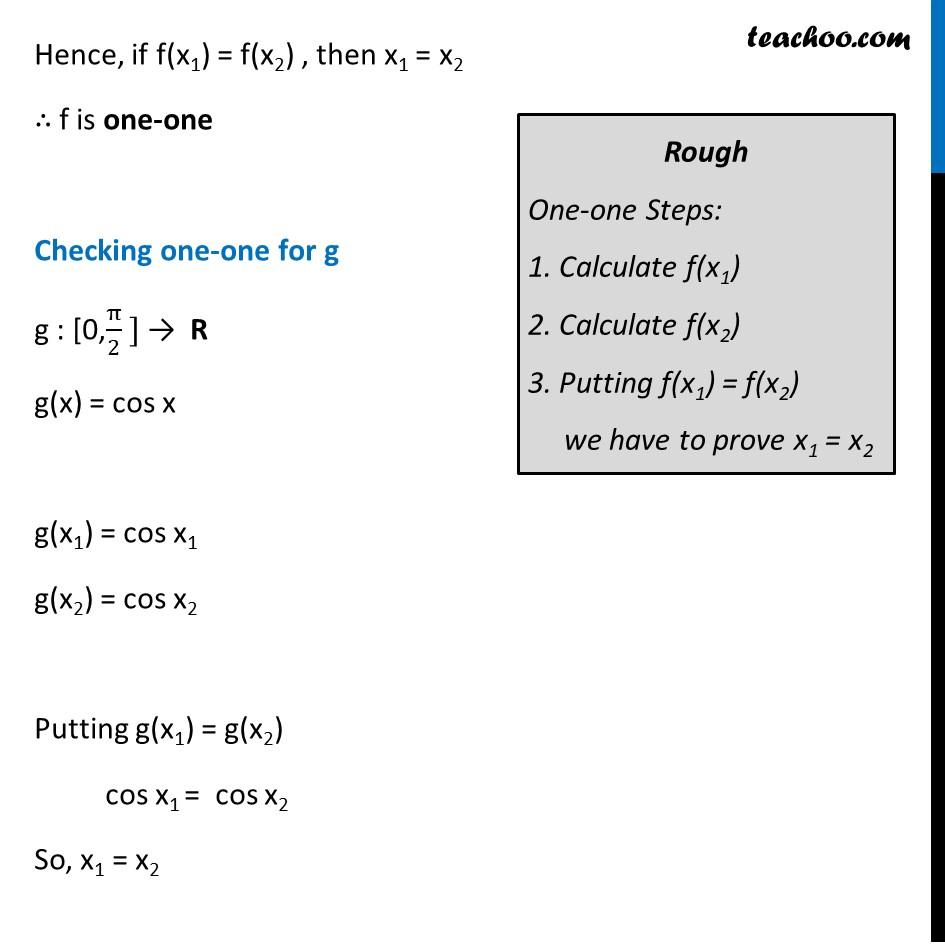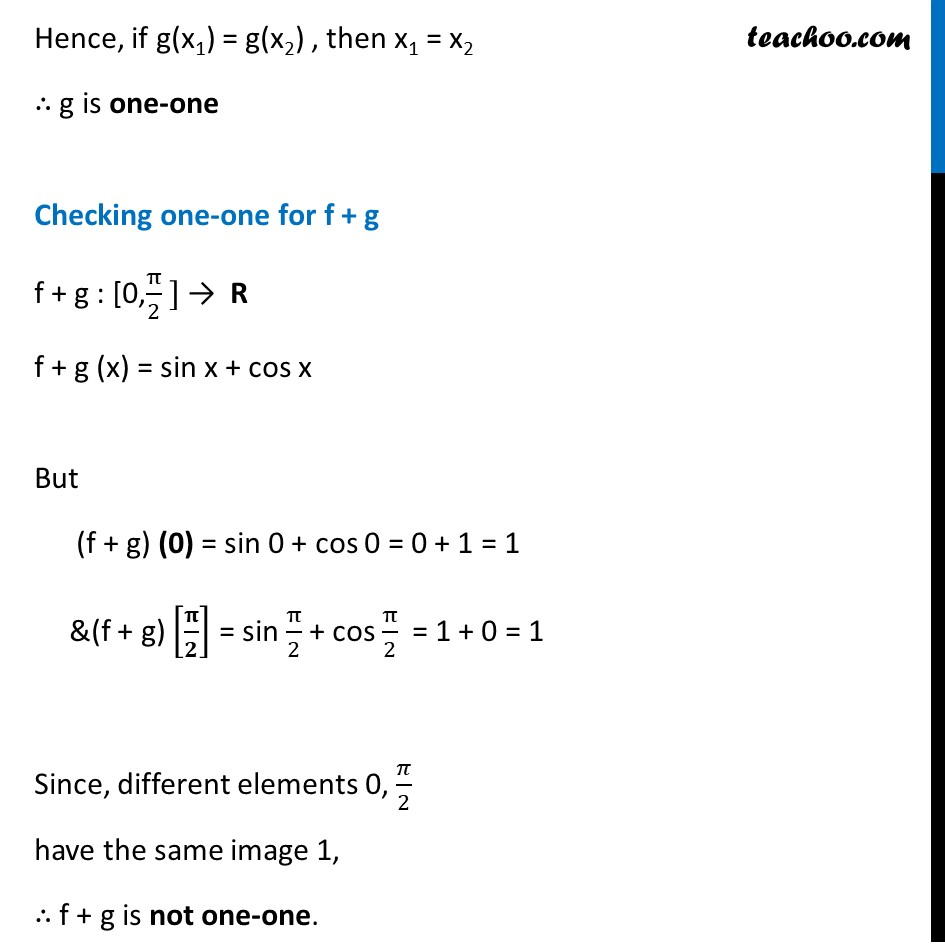To prove one-one & onto (injective, surjective, bijective)

Chapter 1 Class 12 Relation and Functions
Concept wiseLearn in your speed, with individual attention - Teachoo Maths 1-on-1 Class

### Transcript

Example 26 Consider a function f : [0,π/2 ] → R given by f (x) = sin x and g: [0,π/2 ] → R given by g(x) = cos x. Show that f & g are one-one, but f + g is not Checking one-one for f f : [0, π/2 ] → R f (x) = sin x f(x1) = sin x1 f(x2) = sin x2 Putting f(x1) = f(x2) sin x1 = sin x2 So, x1 = x2 Rough One-one Steps: 1. Calculate f(x1) 2. Calculate f(x2) 3. Putting f(x1) = f(x2) we have to prove x1 = x2 Hence, if f(x1) = f(x2) , then x1 = x2 ∴ f is one-one Checking one-one for g g : [0,π/2 ] → R g(x) = cos x g(x1) = cos x1 g(x2) = cos x2 Putting g(x1) = g(x2) cos x1 = cos x2 So, x1 = x2 Hence, if g(x1) = g(x2) , then x1 = x2 ∴ g is one-one Checking one-one for f + g f + g : [0,π/2 ] → R f + g (x) = sin x + cos x But (f + g) (0) = sin 0 + cos 0 = 0 + 1 = 1 &(f + g) [𝛑/𝟐] = sin π/2 + cos π/2 = 1 + 0 = 1 Since, different elements 0, 𝜋/2 have the same image 1, ∴ f + g is not one-one.